Click to Chat

1800-1023-196

+91-120-4616500

CART 0

• 0

MY CART (5)

Use Coupon: CART20 and get 20% off on all online Study Material

ITEM
DETAILS
MRP
DISCOUNT
FINAL PRICE
Total Price: Rs.

There are no items in this cart.
Continue Shopping```Trigonometric Functions

Table of Content

Some Basic Results

Trigonometric Ratios of any Angle

Basic Formulae

In a right angled triangle ABC, ∠CAB = A and ∠BCA = 90° = p/2. AC is the base, BC the altitude and AB is the hypotenuse. We refer to the base as the adjacent side and to the altitude as the opposite side. There are six trigonometric ratios, also called trigonometric functions or circular functions. With reference to angle A, the six ratios are: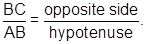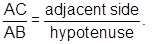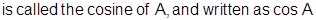Obviously,.

The reciprocals of sine, cosine and tangent are called the cosecant, secant and cotangent of A respectively. We write these as cosec A, sec A, cot A respectively.

Important Notes:

Since the hypotenuse is the greatest side in a right angle triangle, sin A and cos A can never be greater than unity and cosec A and sec A can never be less than unity.

All the six trigonometric functions have got a very important property in common that is periodicity. Remember that the trigonometrical ratios are real numbers and remain same as long as the angle remains same.

Some Basic Results

cos2A + sin2A = 1

From this formula, we can also derive cos2A = 1 - sin2A or sin2A = 1 - cos2A

1 + tan2A = sec2A

So, from this it follows that sec2A - tan2A = 1

cot2A + 1 = cosec2A  or  cosec2A - cot2A = 1

Fundamental inequalities: For 0 < A <, 0 < cosA <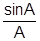<.
It is possible to express trigonometrical ratios in terms of any one of them

e.g.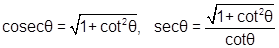Trigonometric Ratios of any Angle

Consider the system of rectangular co-ordinate axes dividing the plane into four quadrants. A line OP makes angle q with the positive x-axis. The angle q is said to be positive if measured in counter clockwise direction from the positive x-axis and is negative if measured in clockwise direction. The positive values of the trigonometric ratios in the various quadrants are shown, the signs of the other ratios may be derived. Note that ∠xoy = p/2, ∠xox' = p , ∠xoy' = 3p/2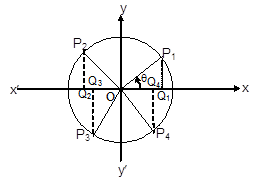PiQi is positive if above the x-axis, negative if below the x-axis, OPi is always taken positive. OQi is positive if along positive x-axis, negative if in opposite direction.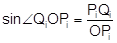(Where i = 1, 2, 3, 4)

Thus depending on signs of OQi and PiQi the various trigonometrical ratios will have different signs.

TABLE

a equals

sin a

cos a

tan a

cot a

Sec a

cosec a

–q

– sinq

cosq

–tanq

– cotq

secq

–cosec q

90° – q

cosq

sinq

cotq

tanq

cosecq

secq

90° + q

cosq

– sinq

–cotq

– tanq

–cosecq

secq

180°– q

sinq

– cosq

– tanq

– cotq

– secq

cosecq

180°+ q

– sinq

– cosq

tanq

cotq

– secq

–cosecq

360°– q

– sinq

cosq

– tanq

– cotq

secq

–cosecq

360°+ q

sinq

cosq

tanq

cotq

secq

cosecq

Note:

Angle q and 90°–q are complementary angles, q and 180°–q are supplementary angles
sin(np + (–1)nq) = sinq, n ÎI
cos(2np ± q) = cosq, n ÎI
tan(np + q) = tanq,  n ÎI

i.e. sine of general angle of the form np + (–1)nq will have same sign as that of sine of angle q and so on. The same is true for the respective reciprocal functions also.

Basic Formulae

Table 1

sin (A + B) = sin A cos B + cos A sin B
sin (A – B) = sin A cos B – cos A sin B
cos (A + B) = cos A cos B – sin A sin B
cos (A – B) = cos A cos B + sin A sin B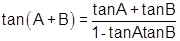sin (A + B) sin (A – B) = sin2 A – sin2 B = cos2 B – cos2 A
cos (A + B) cos (A – B) = cos2 A – sin2 B = cos2 B – sin2 A
sin2A = 2sinA cosA =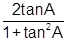cos2A = cos2A - sin2A = 1-2 sin2A = 2cos2A-1 =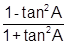tan2A =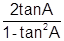sin3A = 3sinA - 4sin3A = 4sin(60° - A) sinAsin(60° + A)
cos3A = 4cos3A - 3cosA = 4cos(60° - A) cosAcos(60°+A)Table 2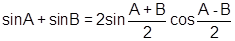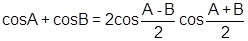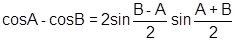tanA + tanB =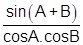2sinAcosB = sin(A + B) + sin (A - B)
2cosAsinB = sin(A + B) - sin (A - B)
2cosAcosB = cos(A + B) + cos(A - B)
2sinAsinB = cos(A - B) - cos (A + B)

Illustration : If in a DABC, cos3A + cos3B + cos3C = 3cosA cosB cosC, then prove that the triangle is equilateral.

Solution:  Given that cos3A + cos3B + cos3C – 3cosA cosB cosC = 0

Þ (cosA + cosB + cosC) (cos2A + cos2B + cos2C – cosAcosB – cosB cosC – cosCcosA) = 0

Þ cos2A + cos2B + cos2C – cosAcosB – cosBcosC – cosCcosA = 0

(as cosA + cosB + cosC = 1 + 4 sinA/2 sinB/2 sinC/2 ¹ 0)

Þ (cosA – cosB)2 + (cosB – cosC)2 + (cosC – cosA)2 = 0

Þ cosA = cosB = cosC

Þ A = B = C, Q 0 < A, B, C < p.

ÞDABC is equilatral.

Illustration:        If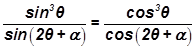, prove that tan2q = 2tan(3q + a).

Solution: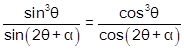= k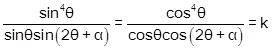==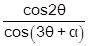Again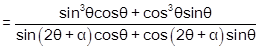=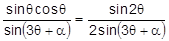\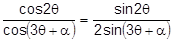=\ tan2q = 2 tan(3q + a).

Illustration :  For  any  real q , find the  maximum value  of  cos2( cosq)  + sin2(sinq) .

Solution:  The  maximum  value of  cos2( cosq) is  1  and  that  of  sin2( sinq) is  sin21, both exists   for  q = p/2. Hence maximum value is 1+ sin21.

Illustration 5:  If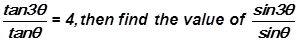.

Solution: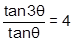Þ tan2q = 1/11

now,= 3 – 4 sin2q =  3 - 4Illustration :  If A, B,C and D are angles of a quadrilateral and sin A/2  sin  B/2. sin C/2. sin D/2 = prove that A = B = C = D = π/2.

Solution:   Given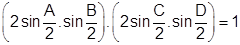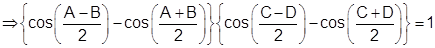Since, A + B = 2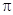- (C + D), the above equation becomes,= 1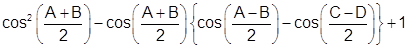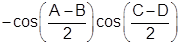= 0.

This is quadratic equation in cos which has real roots.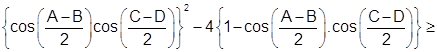0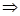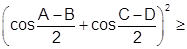4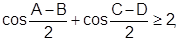Now both cos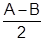and cos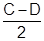1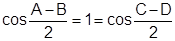A = B, C = D.

Similarly, A = C, B = D  A = B = C = D =/2.

Illustration : If A, B and C are angles of a triangle, prove thatSolution:  Since  A + B + C ==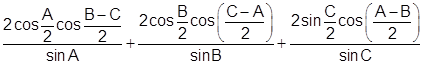=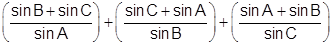=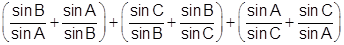as A, B, C are angles of 0 < A, B, C <sin A, sin B, sin C > 0

E2 + 2 + 2E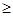6

Watch this Video for more reference

Illustration :  If cos(A + B) sin (C + D) = cos(A – B) sin(C – D),

prove that cotA cotB cotC = cotD.

Solution: We have cos (A + B) sin(C + D) = cos(A – B) sin(C – D)

i.e.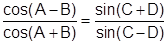orcotA cotB = tanC cotD

or cotA cotB cotC = cotD.

Illustration :  Show that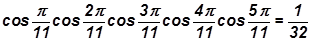Solution:LHS =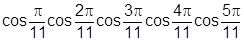Let= a

= cos a cos 2a cos 3a cos 4a cos 5a

= – cos a cos 2a cos 4a cos 8a cos 5a

= – cos 20a cos 21a cos 22a cos 23a cos 5a

= –cos 5a = –=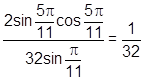.

Illustration : Prove that cot 7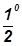=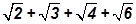Solution:  Let q = 7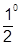Þ 2q = 150

Now cot q ==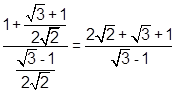=.

For more, you can also refer the papers of previous years.

Illustration :   If 2tan2 a tan2 b tan2 g + tan2 a tan2 b + tan2 b tan2 g + tan2 g tan2 a = 1, prove that sin2 a + sin2 b + sin2 g = 1.

Solution: ÞWe have, 2tan2a tan2btan2g + tan2atan2b + tan2btan2g + tan2gtan2a = 1

Þ 2 + cot2g + cot2a + cot2b = cot2a cot2b× cot2g

Þ cosec2a + cosec2b + cosec2g – 1

= (cosec2a – 1) (cosec2b – 1) (cosec2g – 1)

Þ cosec2a + cosec2b + cosec2g – 1

= – 1 + cosec2a + cosec2b + cosec2g – (cosec2a cosec2b + cosec2b cosec2g  + cosec2gcosec2a + cosec2a× cosec2b× cosec2g

cosec2a cosec2b + cosec2b× cosec2g + cosec2g cosec2a

= cosec2a cosec2b× cosec2gÞ sin2a + sin2b + sin2g = 1
```### Course Features

• 731 Video Lectures
• Revision Notes
• Previous Year Papers
• Mind Map
• Study Planner
• NCERT Solutions
• Discussion Forum
• Test paper with Video Solution